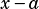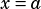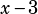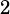Hi Mike! In SAT official test 1, Q 29 (in the math calculator section), how do you solve this problem? I don’t quite understand the explanation/concepts mentioned in the book.

29)For a polynomial p(x), the value of p(3) is -2. Which of the following must be true about p(x)?

A) x-5 is a factor of p(x).
B) x-2 is a factor of p(x).
C) x+2 is a factor of p(x).
D) The remainder when p(x) is divided by x-3 is -2.

(Also what does PWN stand for?)

You can kinda get this by elimination: knowing only thatcan’t possibly tell you anything about any factors of, so you can eliminate A, B, and C, leaving only D as a possible answer.

But why is D right, though? The reason is the polynomial remainder theorem. Most folks remember the most important part of it, which is that if a polynomial is divided byand the remainder is zero, thenis a factor of the polynomial. But the other thing the remainder theorem tells us is exactly what this question is getting at: if a polynomial is divided byand the remainder is anything other than zero, then the remainder equals the polynomial evaluated forChoice D is just stating the remainder theorem: if some polynomialis divided byand the remainder is, thenPWN doesn’t stand for anything, it’s just an old gaming reference.